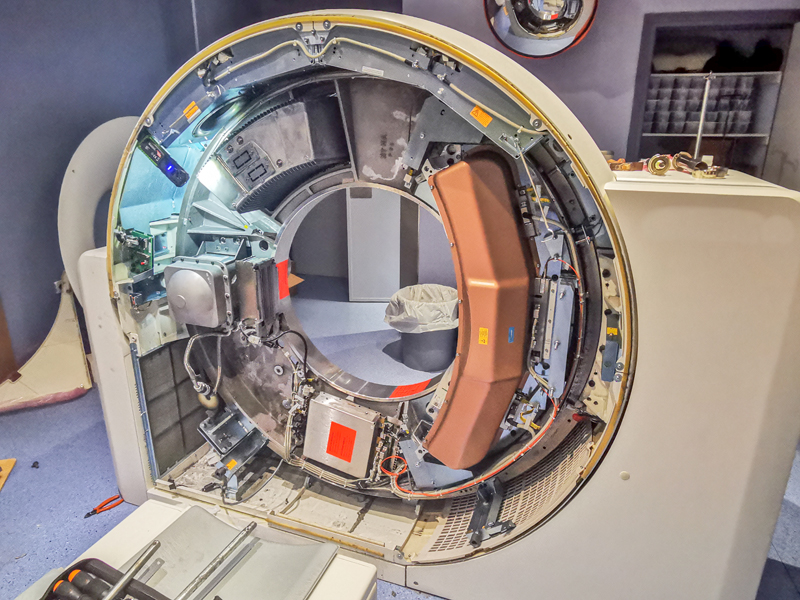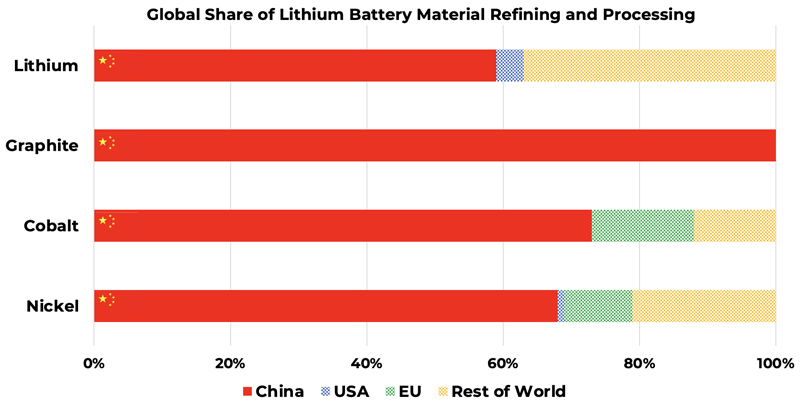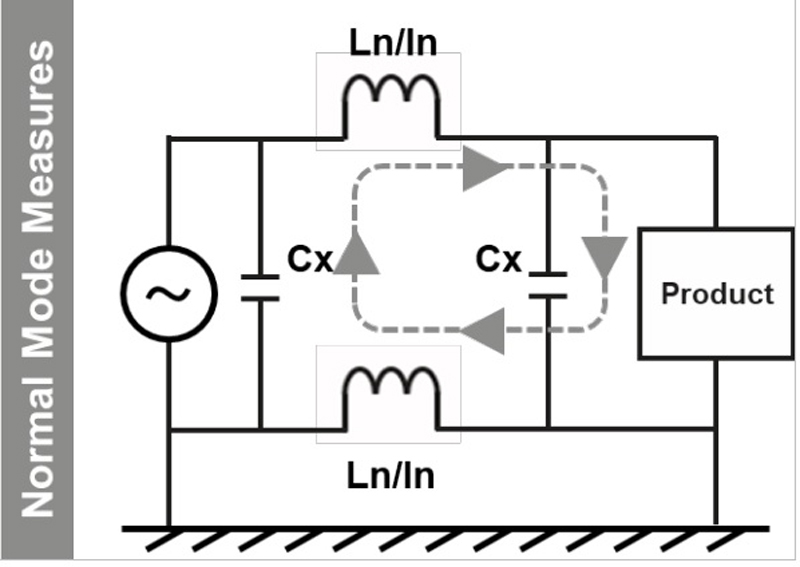# A Smart Solution for Smart Meter Power-Path Management

Author:
Dan Tooth, Texas Instruments

Date
04/10/2018PDF
##### A dual, smart LDO can be used to provide supercap charging and to power a load in smart meter applications

Some smart meter applications require an external wireless communication module to be attached to them. The power requirements imposed are that the module should limit its supply current to be within a 100 – 150mA range. There is also a requirement for a super capacitor (supercap) backup supply to continue powering the module for a period of time after the main power fails. This article addresses how to achieve these requirements.

TPS7B7702-Q1 Dual Linear Regulator (LDO)

TPS7B7702-Q1 is a dual-channel LDO with independent programmable current limits for each channel. The channels are notated as x = 1, 2. The current limit for each channel is set using a resistor from each channel’s ILIMx pin to ground and is calculated by the equation RLIMx = 1.223V x 198 / ILIMx. TPS7B7702-Q1 also provides VSENSEx output voltage signals per channel, proportional to the load currents and governed by the equation VSENSEx = RSENSEx x IOUTx  / 198. Each channel can be configured in either linear regulator (LDO) mode (using a resistor divider from OUTx to FBx to ground) or in load switch mode (FBx tied low). In load switch mode, the input voltage VIN is switched through to OUTx when ENx is high, minus the on-resistance voltage drop of the series MOSFETs.

TPS7B7702-Q1 also has reverse-current blocking fault protection. This fault condition can be detected in two ways by the IC. (1) On the rising edge of ENx, then it checks the OUTx – VIN difference and if it is greater than 500mV(max) it disables the channel and reverse current is blocked. (2) After a channel has been enabled successfully, then if the OUTx is shorted to a voltage greater than VIN and reverse current flows, that channel will turn off its MOSFETs to block the current. Both of these modes are subject to a blanking time of 16ms, whereby the reverse current is first blocked and then latched off after 16ms. The latched-off fault condition can be reset by taking the ENx pin low and then high again, of the affected channel(s).

Figure 1 shows the circuit schematic. CH1 is configured as a load switch (FB1 = gnd) and its current-limit is set by R1+R3. CH2 is configured in LDO mode with a 5V output voltage set by Rfb1 and Rfb2 and with its current-limit set by R1+R2. Connected to the output of CH2 is a supercap and its voltage (VOUT2) is OR’d with CH1’s output voltage (VOUT1) via a diode. The final output to the load (the comm’s module) is either the switched input voltage from CH1, or the supercap voltage. With VOUT1 > VOUT2 - VF then the output voltage is VOUT1.

When CH2 is enabled it enters current-limit mode and charges the supercap with a current given by ILIM2 = 1.223 x 198 / (R1+R2). When in current-limit mode the ERR/ flag pin is low, but goes high after current-limit mode is exited. The current-limit of CH1 is given by ILIM1 = 1.223 x 198 / (R1+R3). Because R1 is common to CH1 and CH2, the total input current drawn from the IN pin can be controlled because the current flowing in one channel influences the current-limit of the other. Figure 2 shows the measured current taken by the load and by the supercap constant current charger when both channels are enabled. For these tests then R1 = 1k5, R2 = 2k5 and R3 = 500Ω. Two limiting cases are; (1) when the supercap has finished charging (OUT2 = 5V) and CH2’s current drops to zero. Maximum current can flow into the load from OUT1 given by 1.223 x 198 / (1k5 + 500Ω) = 121mA. (2) When the load current is zero then the maximum charge current into the supercap from CH2 is 1.223 x 198 / (1k5 + 2k5) = 60mA. Figure 2 shows that when the load current increases above zero then the charge current is reduced.

Click image to enlarge

Figure 2. Variation of the supercap charge current as the load current changes

Thermal considerations impose a maximum limit on the allowable supercap charge current. When the supercap is uncharged and the load is zero then VIN x 60mA = 0.72W is dissipated in TPS7B7702-Q1 when VIN = 12V.

The configuration of the two channels is such that the load is dominant and is supported at the expense of the supercap charge current. Also, the max charge current is limited to prevent thermal issues. The total max input current is a few mA higher than the current calculated when the charge current is zero (121mA). The actual max current can be checked by adjusting the load current around its maximum and observing the total current drawn from the supply.

Figure 3 shows some waveforms without TPS3710 present. Instead of the supercap, a 1000μF electrolytic capacitor is used as it more readily shows the charging / discharging behaviour over a shorter time period. Also from a safety aspect, it is best to experiment without the supercap at first. Wearing eye protection is recommended, as there is a lot of stored energy, especially in the supercap when it is used (~31 Joules). When the 12V power supply is turned on, the load voltage quickly ramps to 12V minus the FET on-resistance drop. The load current is 104mA, which means the charge current is 20mA and this ramps the supercap voltage linearly to 5V. About 0.5 seconds later, the input voltage is manually reduced step-by-step until it falls below the supercap voltage and the supercap discharges into the load. When the supercap voltage and VIN are equal then VIN resumes supporting the load as CH1 has not turned off. The input voltage is then manually recovered back to 12V, but the supercap does not resume charging because the reverse protection of CH2 had been triggered when the supercap voltage was higher than VIN. To resume charging, the EN2 pin is toggled low then high and the supercap charges back up to 5V at time equals 7.5 seconds. It is worth noting that the reverse current protection of CH1 was not triggered during this process and so while the supercap was supplying the load, reverse current occurred through CH1 which raised the input voltage to the supercap voltage. The next section explains how to prevent this and how to avoid the need to toggle EN2 when the input supply returns.

Click image to enlarge

Figure 3. OUT1 (red, 4V/div) and Supercap voltage (blue, 2V/div) during charge / discharge

Figure 1 also shows the TPS3710 voltage supervisor, which is implemented as a comparator with hysteresis. It is also available as an automotive-qualified version TPS3710-Q1. There is also a higher voltage rated device, TPS3711. The IC’s internal voltage reference is VREF = 0.4V. The values of the resistors R4 to R7 shown in Figure 1 means that when VIN ≥ 7V the ENx pins are pulled high and when VIN ≤ 6V they are pulled low. To calculate the resistor values for these “start” and “stop” voltages then let R4 >> R7 and R4 = R5. The values chosen are R4 = 100k and R7 = 2k and R6 and R5 are calculated from the following equations.

Vstart ≈ VREF (R6 + R7) / R7 and Vstop ≈ VREF ([R4 + R5] || R6) + R7) / R7

Figure 4 shows the behavior when TPS3710 is fitted. TPS7B7702-Q1 is disabled when VIN falls below 6V and the capacitor supplies the load (1000μF tested here). When VIN rises above 7V it is re-enabled and both outputs are active again. CH1 supports the load and CH2 recharges the capacitor. When TPS7B7702-Q is disabled, it is blocking reverse current.

Click image to enlarge

Figure 4. With TPS3710 fitted, OUT1 (red, 4V/div) and Supercap voltage (blue, 2V/div) during charge / discharge and auto-recover

Texas Instruments

### RELATED#### Patient Health Through System Health

Aug 1,2022#### A Battery Designed for Energy Storage

Aug 1,2022#### Compact Filter Saves Space in Three-Phase Power Applications

Aug 1,2022Aug 1,2022